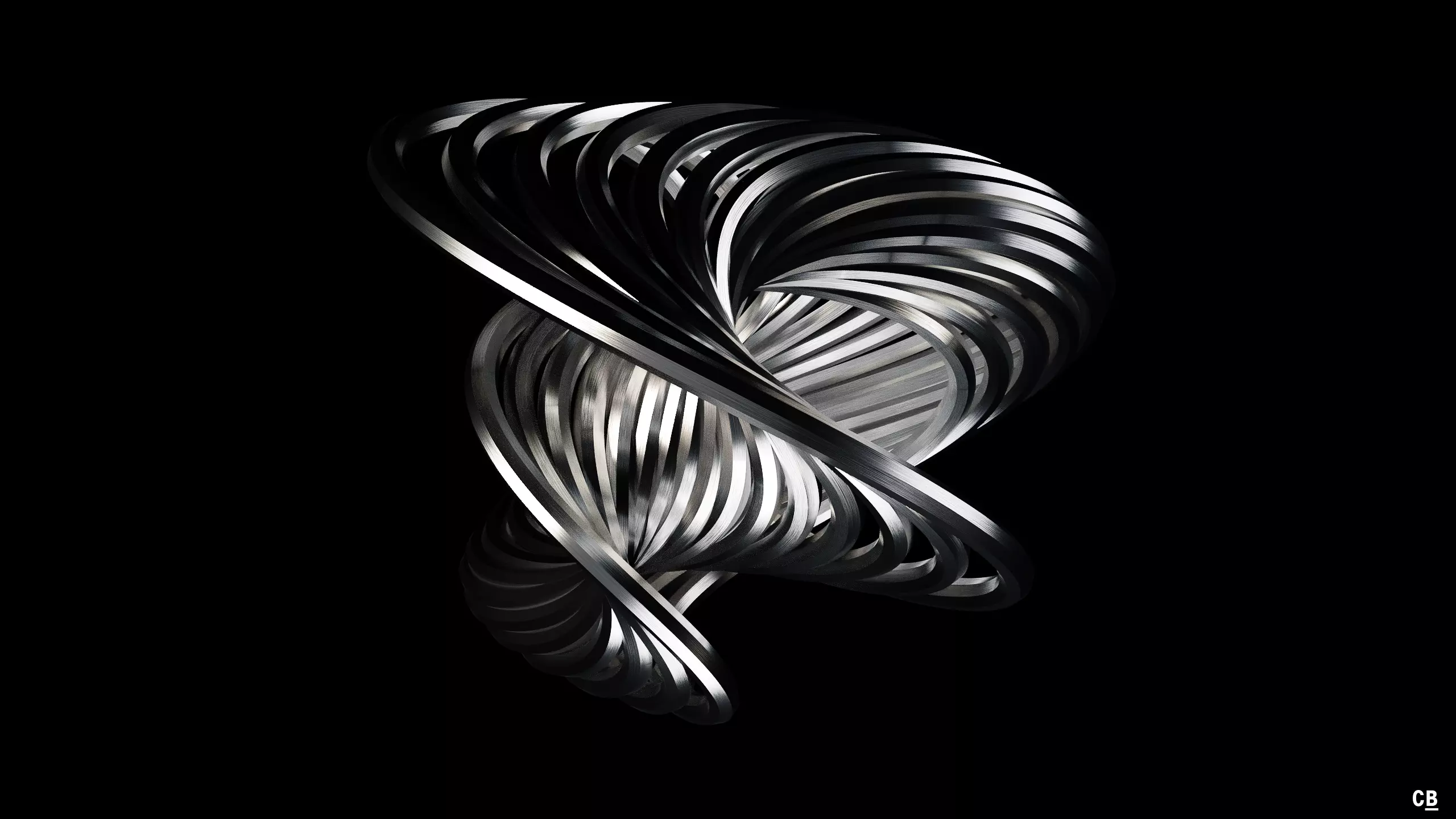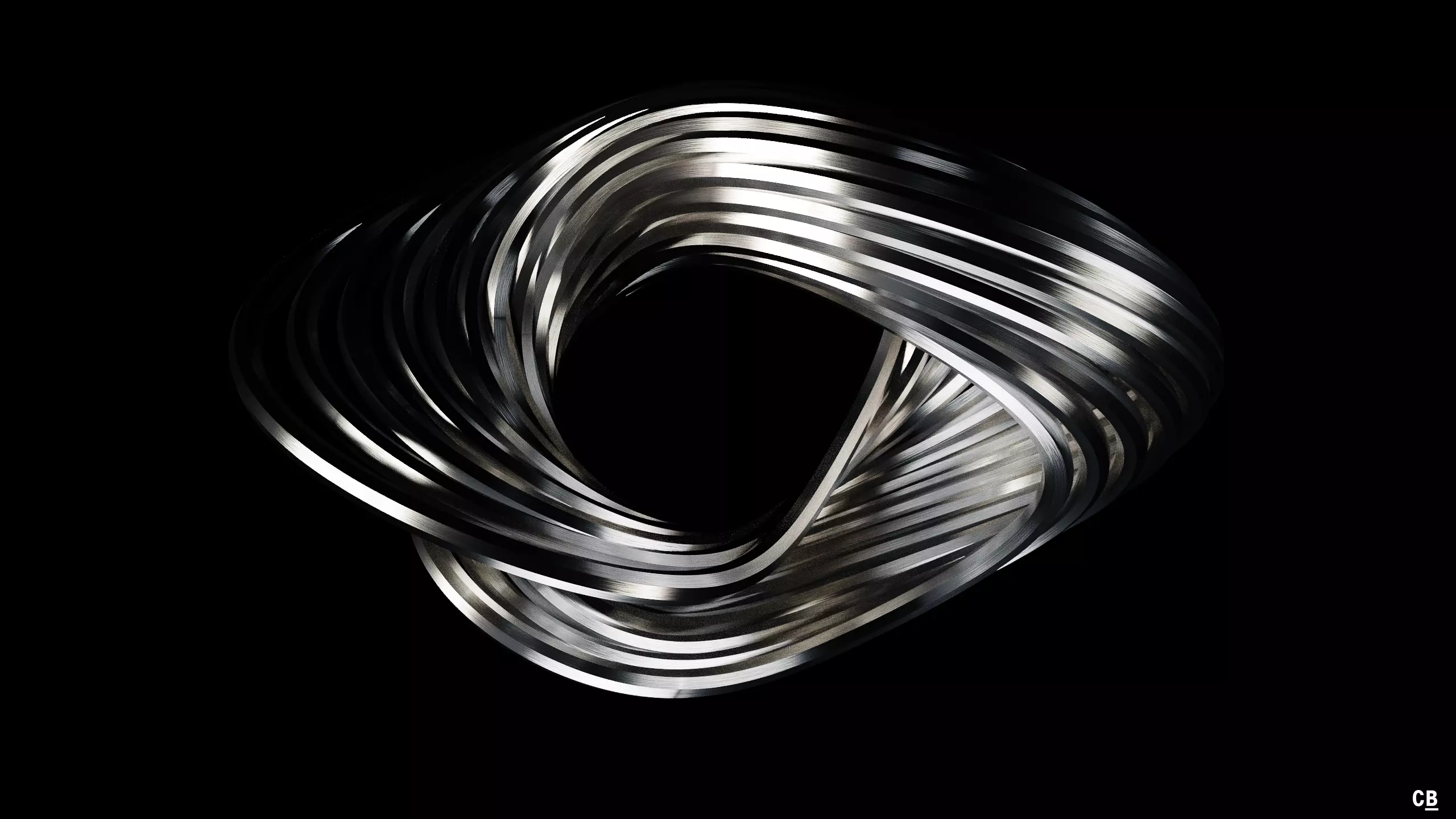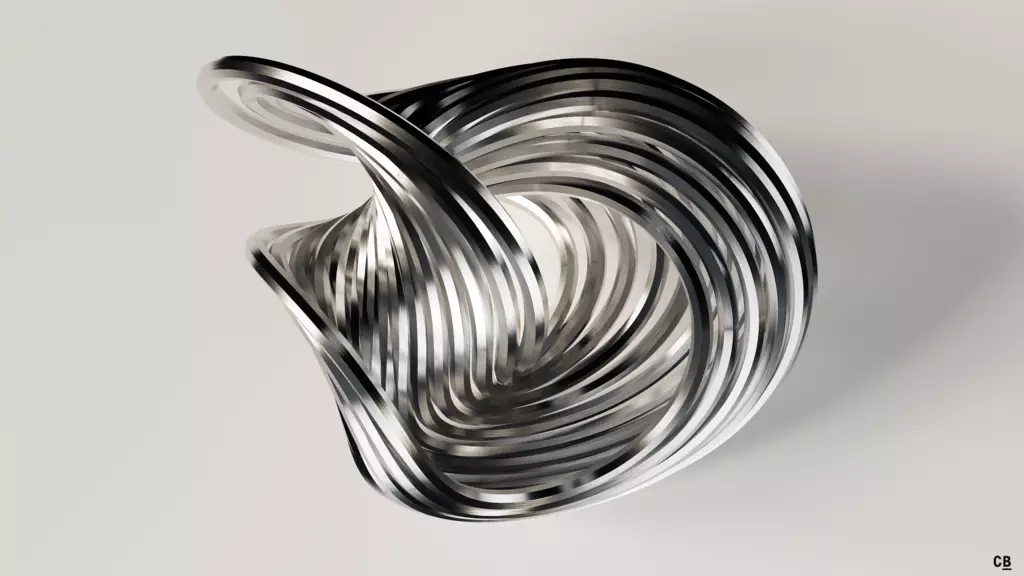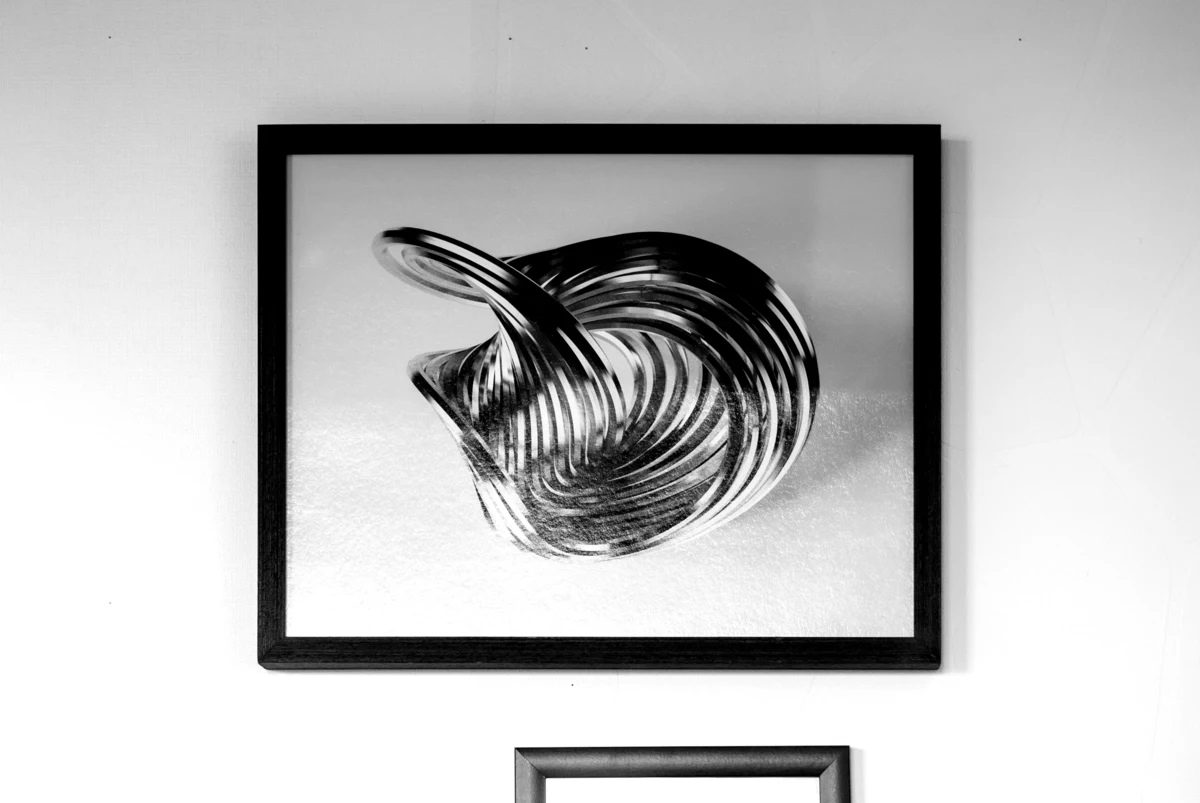# Nosé-Hoover Attractor

This set of dynamical equations was found by Shūichi Nosé in 1984 when researching about molecular dynamics and thermal equilibrium distribution1:

$\dot{q} = \frac{p}{s^2}$ $\dot{p} = -q$ $\dot{s} = \zeta$ $\dot{\zeta} = \frac{p^2}{s^3} - \frac{T}{s}$

Further research by William Graham Hoover2 a year later simplified them to3:

$\dot{q} = p$ $\dot{p} = -q - \zeta\, p$ $\dot{\zeta} = p^2 - T$

## Renders

With $$T = 1.5$$:## Framing It

I liked the third render so much, that I printed it on 45×35 cm fine-art paper and framed it. Read more in my blog post.## Stereographic Animation

1. S. Nosé, 1984. "A unified formulation of the constant temperature molecular dynamics methods". J. Chem. Phys. 81(1). doi:10.1063/1.447334

2. Professor Doctor Hoover has a great homepage where you can find all of his research, books and lectures.

3. W.G. Hoover, 1985. "Canonical dynamics: Equilibrium phase-space distribution". Phys. Rev. A. 31(3). doi:10.1103/PhysRevA.31.1695

🕸 💍